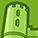Announcements

#### Curious on how you can apply MOSFET to Smart Home Appliances? Register for the ASEAN Smart Home Appliance Webinar here to find out how.

Tip / Sign in to post questions, reply, level up, and achieve exciting badges. Know more

# MOSFET (Si/SiC) Forum DiscussionsLevel 2Level 2

# Co(tr) only for WBG-Transistors?

Hello,

I was wondering if the Co(tr) is also available for other FETs/Transistors than GaN and SiC.

Additionally, I was wondering if Co(tr) can also be used for voltage levels, which are different to the voltage level used to determine Co(tr). For example, Co(tr) was determined by loading the output capacitance from 0 to 500V, but the voltage level used is only 300V.

This is my understanding of Co(tr):
The fixed capacitance Co(tr) is used to capture the relationship between charge and voltage of the discontinuous output capacitance. Here, the equivalent capacitance Co(tr) is determined by charging the output capacitance at a current i and a voltage V, and thus describes the time-related effective output capacitance. Thus, Co(tr) can be used to determine the charge/discharge time of the output capacitance Coss independent of the topology.

Best regards

1 SolutionModeratorModerator

# Re: Co(tr) only for WBG-Transistors?

Hi there,

Yes. Irrespective of FET type, concept can be implemented for all types of FETs.

For 300V also Co(tr) could be derived using the relation given in the below thread.  Please use Vds as 300V.

https://community.infineon.com/t5/MOSFET-Si-SiC/Co-er-vs-Co-tr/td-p/358950

8 RepliesModeratorModerator

# Re: Co(tr) only for WBG-Transistors?

Hi there,

Yes. Irrespective of FET type, concept can be implemented for all types of FETs.

For 300V also Co(tr) could be derived using the relation given in the below thread.  Please use Vds as 300V.

https://community.infineon.com/t5/MOSFET-Si-SiC/Co-er-vs-Co-tr/td-p/358950Level 2Level 2

# Re: Co(tr) only for WBG-Transistors?

Hi,

The concept of Co(tr) is normally not used for other FET as long as the Coss is continuous and linear. Right?

To derive the Co(tr) with this formula, a measurement must be done to get the values for the time and current. So it can not be simply converted to another voltage level directly. Or is it possible to convert it directly?ModeratorModerator

# Re: Co(tr) only for WBG-Transistors?

Hi there,

approximation can be done to linear above 50V (assumed value however nearby) Vds.

you can check di/dt of magnetizing inductance current to get the idea of timings and current using simulation in Ltspice preferably, as Lm is in series with FETs. testing is not necessary simulation is far sufficient.ModeratorModerator

# Re: Co(tr) only for WBG-Transistors?

also you can check individual mosfet datasheet Coss characteristics to understand the linearization of voltage levels, for eg. 50VLevel 2Level 2

# Re: Co(tr) only for WBG-Transistors?

The output capacitance Coss is dynamic  and changes normally nonlinearly as Vds changes.
The concept of the time related effective output capacitance Co(tr) approximates the Coss to a linear value.
So the Co(tr) must be calculated for the desired voltage level where the FET is being used.

And therefor linear equations can be used with Co(tr), e.g.: V=1/C*Q

Is this correct?

What is the reason why approximation can be done above the assumed value 50V Vds (nearby)? Is it due to the large drop (step change) of the value of Coss before that voltage level?ModeratorModerator

# Re: Co(tr) only for WBG-Transistors?

hi,

you can check the characteristics of Coss in datasheet of any mosfet you will find the linearity in the curve, the thing is, the line is not always straight  so you have to adjust some sampling points in order to assume linear.  for equation you can consider the above link.Level 2Level 2

# Re: Co(tr) only for WBG-Transistors?

Regarding the benefit of using the time related effective output capacitance Co(tr)...

The effective capacitance is all capacitance added up (or the average of them?). This can't be easily done for the output capacitance Coss due to the nonlinear (discontinuous?) properties. Coss is a Function of the drain to source voltage Vds.

The time related effective output capacitance Co(tr) gives a fixed capacitance that would give the same charging time as the output capacitance Coss. So it's easier to determine Co(tr).

Can one confirm my thought?ModeratorModerator

# Re: Co(tr) only for WBG-Transistors?